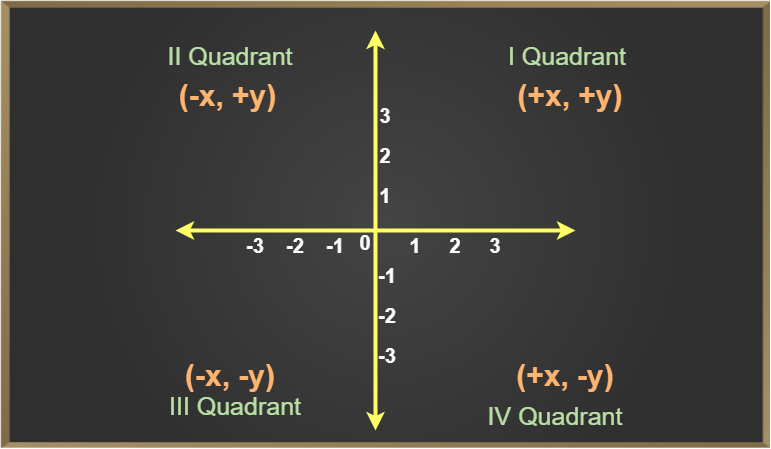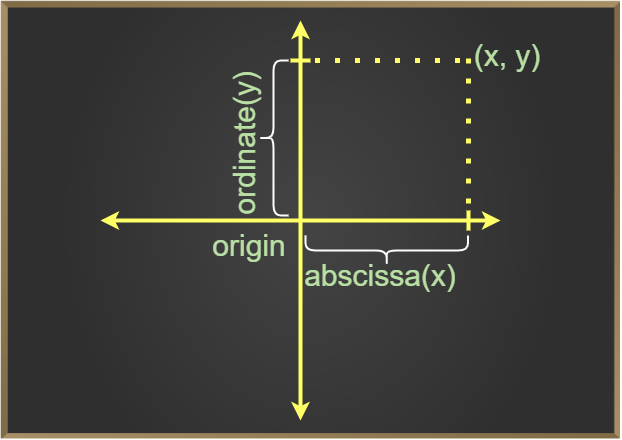GeeksforGeeks App
Open AppBrowser
Continue

## Related Articles

Coordinate geometry is the branch of mathematics that deals with plotting the curve on the coordinate axes. Various curves can be plotted on the coordinate plane using coordinate geometry formulas. Co-ordinate geometry uses algebraic equations to plot various curves on the coordinate plane. One of the popular coordinate systems used in mathematics is the rectangular Cartesian system.

## What is Coordinate Geometry?

Coordinate geometry is a branch of mathematics, which is used to represent various geometric figures in a 2-D plane. Coordinate geometry is used to describe various curves such as circles, parabolas, etc. Coordinate geometry divides the coordinate plane into four different quadrants, which are the I quadrant, II quadrant, III quadrant, and IV quadrants.## Coordinates of a Point

A coordinate is an address, which helps to locate a point in space. For a two-dimensional space, the coordinates of a point are (x, y). Here let us take note of these two important terms.

Abscissa: It is the x value in the point (x, y), and is the distance of this point along the x-axis, from the origin
Ordinate:  It is the y value in the point (x, y)., and is the perpendicular distance of the point from the x-axis, which is parallel to the y-axis.
The coordinates of a point are useful to perform numerous operations of finding distance, midpoint, the slope of a line, and equation of a line.

The coordinates of a point are the real variables associated in an order to describe its location in space. Here we consider the space to be two-dimensional. Let’s have a look at the following terms.

• Origin: Through a point O, referred to as the origin, we take two mutually perpendicular lines XOX’ and YOY’, and call them x and y axes respectively.
• Abscissa & Ordinate: The position of a point is completely determined with reference to these axes by means of an ordered pair of real numbers (x, y) called the coordinates of P where | x | and | y | are the distances of the point P from the y-axis and the x-axis respectively, x is called the x-coordinate or the abscissa of P and y is called the y-coordinate or the ordinate of the point P.## Coordinate Geometry Formulas

The formulas of coordinate geometry help in conveniently proving the various properties of lines and figures represented in the coordinate axes. The formulas of coordinate geometry are the distance formula, slope formula, midpoint formula, section formula, and the equation of a line. Let us know more about each of the formulas in the below paragraphs.

## Distance Formula

The distance between two points A(x1, y1) and B(x2, y2) is given by

AB = √((x2 – x1)2 + (y2 – y1)2)

The distance of the point P(x, y) from the origin O(0, 0) is given

OP = √((x – 0)2+ (y – 0)2), i.e. OP = √(x² + y²)

Example: Find the distance between the points P(-4, 7) and Q(2, -5).

Solution:

The given points are P(-4, 7) and Q(2, -5).

Then, (x1 = -4, y1 = 7) and (x2 = 2, y2 = -5). :

PQ =  √((x2 – x1)2 + (y2 – y1)2)

= √((2 – (-4))2 + (-5 – 7)2)

= √(62 + (-12)2

= √(36 + 144)

= √180

= 6√5

## Mid-Point Formula

The midpoint is the point that divides any line segment into two equal parts. The formula to find the midpoint of the line with endpoints A(x1, y1) and B(x2, y2) is given by,

midpoint (x,y) = {(x1+x2)/2 , (y1+y2)/2}

Example: Find the coordinates of the midpoint of the line segment joining points A(-5, 4) and B(7,-8).

Solution:

Let M(x, y) be the midpoint of AB. Then,

x = ((-5) + 7)/2 =1 and  y = ((4 + (-8)) = -2

Hence, the required point is M (1, -2).

## Section Formula

The coordinates of the point P(x, y) which divides the line segment joining A(x1, y1) and B(x2, y2) internally in the ratio m:n are given by

x = (mx2 + nx1) / (m+n)

y = (my2+ny1) / (m+n)

Example: Find the coordinates of the point that divides the line segment joining the points A(5, 4) and B(7,8) in the ratio of 2:3.

Solution:

Let M(x, y) be the required point,

given m:n = 2:3 and (x1, y1) = (5, 4) , (x2, y2) = (7,8)

now for M(x,y)

x = (mx2 + nx1) / (m+n)

= (2×7 + 3×5)/(2 + 3)

= 29/5

y = (my2+ny1) / (m+n)

= (2×8 + 3×4)/(2 + 3)

= 40/5

= 8

So, the required point M(x,y) is (29/5 , 8)

## Slope Formula

The slope of a line is the inclination of the line. Slope Formula is calculated by measuring the angle made by the line with the positive x-axis, or by calculating it using any two points on the line. The slope of a line inclined at an angle θ with the positive x-axis is given by m = tan θ.  The slope of a line joining the two points A(x1, y1) and B(x2, y2) is given by

m = tan θ = (y2-y1) / (x2-x1)

Example: Find the slope of the line segment joining by points A(5, 1) and B(7,8).

Solution:

given,  (x1, y1) = (5, 1) , (x2, y2) = (7, 8)

Slope (m) = (y2-y1) / (x2-x1)

= (8-1)/(7-5)

= 7/2

## Area of Triangle

The area of a triangle ABC with vertices A(x1, y1), B(x2, y2), and C(x3, y3) is given by

area(ABC) = |1/2 {x1(y2 – y3) + x2(y3 – y1) + x3 (y1 – y2)}|

Example: Find the area of the triangle whose vertices are A(2, 7), B(3, -1), and C(-5, 6).

Solution:

Let A(2, 7), B(3, -1) and C(-5, 6) be the vertices of the given △ABC.

Then,

(x1 = 2, y1 = 7), (x2 = 3, y2 = -1) and (x3 = -5, y3 = 6).

Area of △ABC =  |1/2 {x1(y2 – y3) + x2(y3 – y1) + x3 (y1 – y2)}|

= 1/2 |2(-1 – 6) + 3(6 – 7) – 5(7 +1)|

= 1/2| -14 – 3 – 40|

= 1/2|-57|

= 57/2

= 28.5 sq units.

## Condition for Collinearity of Three Points

Let the given points be A(x1, y1), B(x2, y2), and C(x3, y3). Then A, B, and C are collinear,

area of ABC = 0

1/2[x1(y2 – y1) + x2(y3 – y1) + x3(y1 – y2)] = 0

Example: Show that points A(-1, 1), B(5, 7), and C(8,10) are collinear.

Solution:

Let A(-1, 1), B(5, 7) and C(8, 10) be the given points.

Then, (x1 = -1, y1 = 1), (x2 = 5, y2 = 7) and (x3 = 8, y3 = 10)

∴  x1(y2 – y3) + x2(y3 – y1) + x3(y1 – y2)

= (-1) (7 – 10) + 5(10 -1) + 8(1 – 7)

= (3 + 45 – 48)

= 0

Hence, the given points are collinear.

## Centroid of a Triangle

Centroid of a triangle is the point of intersection of all three medians of a triangle. The centroid of a triangle having its vertices A(x1,y1), B(x2,y2), and C(x3,y3) is given by the formula

Centroid (x,y) = {(x1+x2+x3) / 3, (y1+y2+y3) / 3}

Example: Find the centroid of the triangle whose vertices are A(2, 7), B(3, -1), and C(-5, 6).

Solution:

Let A(2, 7), B(3, -1) and C(-5, 6) be the vertices of the given △ABC.

(x1 = 2, y1 = 7), (x2 = 3, y2 = -1) and (x3 = -5, y3 = 6).

Centroid (x,y) = {(x1+x2+x3) / 3 , (y1+y2+y3) / 3}

= {2+3+(-5)}/3 , {7+(-1)+6}/3

= (0 , 12)

## Solved Examples on Coordinate Geometry

Example 1: Show that the points A(a, b + c), B(b, c + a), and C(c, a + b) are collinear.

Solution:

Let A(a, b + c), B(b, c + a) and C(c, a + b) be the given points. 11

Then, (x1 = a, y1 = b + c); (x2 = b, y2 = c + a); and (x3 = c, y3 = a + b).

∴ a(c + a – a – b) + b(a + b – b – c) + c(b + c – c – a)

= a(c – b) + b(a – c)+ c(b – a)

= 0

Hence, the given points are collinear.

Example 2: Find the area of quadrilateral ABCD whose vertices are A(-4, -2), B(-3, -5), C(3, -2), and D(2, 3).

Solution:

Join A and C. Then, area of quad. ABCD = ar(△ABC) + ar(△ACD)

Area of  △ABC = 1/2|{(-4).(-5 + 2) -3(-2 + 2) + 3(-2 + 5)}|

= 21/2 sq units.

Area of  △ACD = 1/2|{ (-4).(-2 – 3) + 3(3 + 2) + 2(-2 + 2}|

= 35/2

Area of quad. ABCD = 21/2 + 35/2  sq units = 28 sq units.

Example 3: Find the coordinates of the points of trisection of the line segment joining the points A(-5, 6) and B(4, -3).

Solution:

Let P and Q be the points of trisection of AB.

Then, P divides AB in the ratio 1 : 2

So, the coordinates of P are

P((1 x 4 + 2 x (-5)/1 + 2, ((1 x (-3) + 2 x 6)/1 + 2) i.e., P(-2, 3).

Also, Q divides AB in the ratio 2:1.

So, the coordinates of Q are

Q((2 x 4 + 1 x (-5)/2 + 1, (2x(-3) +1 x 6/1 + 2)) i.e., Q(1, 0)

Q(1, 0).

Example 4: Find the distance of point P(6, -6) from the origin.

Solution:

Let P(6, -6) be the given point and O(0, 0) be the origin.

Then, OP = √((6 – 0)2 + (-6 – 0)2)

= √(62 + (-6)2)

= √72

= 6√2

## FAQs on Coordinate Geometry

Question 1: What is meant by Cartesian Plane?

The space is assumed to consist of an imaginary plane, to study the coordinate geometry. this imaginary plane is called the Cartesian plane. It is formed by two perpendicular lines the x-axis (horizontal axis) and the y-axis (vertical axis). The position of any point in the Cartesian plane is determined by the ordered pair (x, y).

Question 2: What is the importance of Coordinate Geometry?

Coordinate geometry is of great importance in our life. It is used by us on a daily basis which includes:

• GPS and Map Navigation uses coordinate geometry to mark our location.
• It used by airline industry to plot the trajectory of a plane.
• Latitude and Longitude system uses coordinate geometry to work.
• It is also used for military purposes.

Question 3: What is meant by Abscissa and Ordinates in Coordinate Geometry?

Abscissa and Ordinate represent the position of a point on the plane. Horizontal component or the value at X-axis is called the abscissa while the vertical component or the value at Y-axis is called the ordinate. For example, in the point (7, 9), 7 is the abscissa and 9 is the ordinate.

Question 4: What is the coordinate of a point?